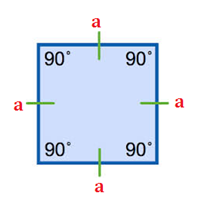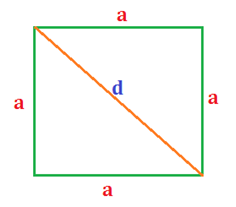# Square Formula

Square is a regular quadrilateral. All the four sides and angles of a square are equal. The four angles are 90 degrees each, that is, right angles. A square may also be considered as a special case of rectangle wherein the two adjacent sides are of equal length.

In this section, we will learn about the square formulas – a list of the formula related to squares which will help you compute its area, perimeter, and length of its diagonals. They are enlisted below:

$$\begin{array}{l}Area\; of\; a\; Square\; = a^{2}\end{array}$$

Perimeter of a Square =4a

$$\begin{array}{l}Diagonal\; of\; a\; Square\; = a\sqrt{2}\end{array}$$

Where ‘a’ is the length of a side of the square.

Properties of a Square

•The lengths of all its four sides are equal.
• The measurements of all its four angles are equal.
• The two diagonals bisect each other at right angles, that is, 90°.
• The opposite sides of a square are both parallel and equal in length.
• The lengths of diagonals of a square are equal.

Derivations:

Consider a square with the lengths of its side and diagonal are a and d units respectively.Formula for area of a square: Area of a square can be defined as the region which is enclosed within its boundary. As we mentioned, a square is nothing a rectangle with its two adjacent sides being equal in length. Hence, we express area as:

The area of a rectangle = Length × Breadth

Here,

$$\begin{array}{l}Area\; of\; square\; = a \times a = a^{2}\end{array}$$

The formula for the perimeter of a square: Perimeter of the square is the length of its boundary. The sum of the length of all sides of a square represents its boundary. Hence, the formula can be given by:

Perimeter = length of 4 sides

Perimeter = a+ a + a + a

Perimeter of square = 4a

The formula for diagonal of a square: A diagonal is a line which joins two opposite sides in a polygon. For calculating the length diagonal of a square, we make use of the Pythagoras Theorem.

In the above figure, the diagonal’ divides the square into two right angled triangles. It can be noted here that since the adjacent sides of a square are equal in length, the right angled triangle is also isosceles with each of its sides being of length ‘a’.

Hence, we can conveniently apply the Pythagorean theorem on these triangles with base and perpendicular being ‘a’ units and hypotenuse being’ units. So we have:

$$\begin{array}{l}d^{2}=a^{2}+a^{2}\end{array}$$

Or

$$\begin{array}{l}d=\sqrt{2a^{2}}=a\sqrt{2}\;units\end{array}$$

Solved examples:

Question 1: A square has one of its sides measuring 23 cm. Calculate its area, perimeter, and length of its diagonal.

Solution: Given,

Side of the square = 23 cm

Area of the square:

Area of the square =

$$\begin{array}{l}a^{2}=23^{2}=529 cm^{2}\end{array}$$

Perimeter of the square:

Perimeter of the square= 4a= 4 × 23 = 92 cm

Diagonal of a square:

Diagonal of a square =

$$\begin{array}{l}a\sqrt{2}=23\sqrt{2\;cm}=32.52cm\end{array}$$

Question 2:  A rectangular floor is 50 m long and 20 m wide. Square tiles, each of 5 m side length, are to be used to cover the floor. Find the total number of tiles which will be required to cover the floor.

Solution: Given,

Length of the floor = 50 m

$$\begin{array}{l}No.\; of\; tiles\; needed = \frac{Area\; of\; floor}{Area\; of\; one\; tile}=\frac{1000}{25}=40\; tiles\end{array}$$